# CBSE Class 12 Physics Notes Chapter 6 Electromagnetic Induction

## Define Magnetic Flux

We can define the magnetic flux of a system through a surface area A and it is placed in a uniform magnetic field B as

$$\begin{array}{l}\phi _{B} = B.A = BA Cos\theta\end{array}$$

The

$$\begin{array}{l}\theta\end{array}$$
represents the angle between A and B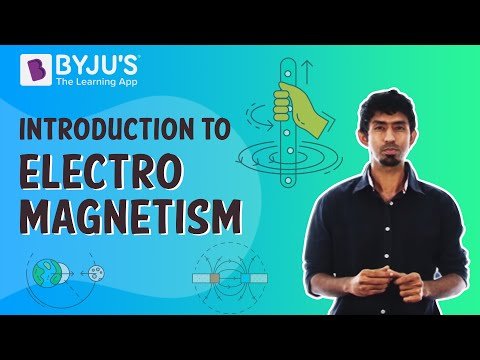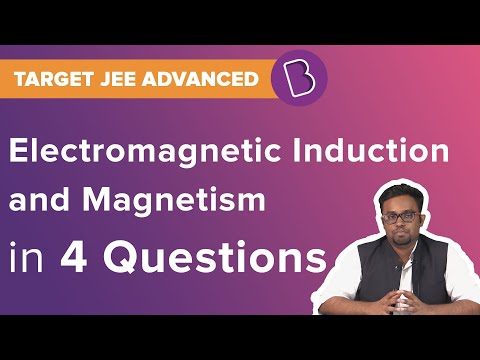According to this law, the emf induced in a coil of N turns is indirectly proportional to the rate of change of flux through it.

$$\begin{array}{l}\varepsilon \, = -N\frac{\mathrm{d} \phi_{B}}{\mathrm{d} t}\end{array}$$

Here the numerator is the flux associated with only one turn of the coil. When the circuit is closed, a current I=

$$\begin{array}{l}\varepsilon\end{array}$$
R is found in it, where R is the resistance of the circuit.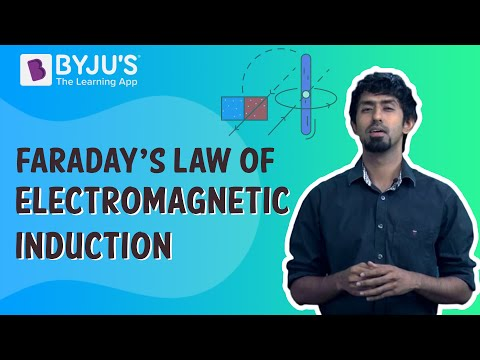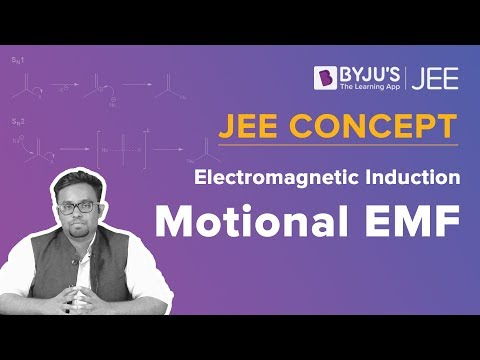### What Is Lenz’s Law?

Lenz’s law states that the polarity of the emf, which is induced, tends to produce a current opposing to the change in the magnetic flux that produced it. The negative sign represents the opposition.

### Eddy Currents

In nearby metal bodies, changing the magnetic fields can create current loops, which get dissipated in the form of heat. These currents are known as Eddy currents.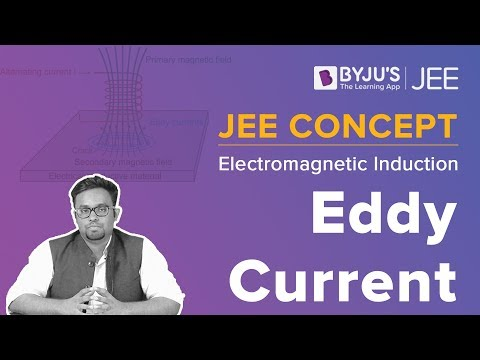### Define Inductance

Inductance is defined as the ratio between the current and the flux-linkage, which is represented by

$$\begin{array}{l}\varepsilon\end{array}$$

Students can refer to the short notes and MCQ questions along with separate solution pdf of this chapter for quick revision from the links below:

To know more about electromagnetic induction, keep visiting BYJU’S.

### Important Questions

1. If the current carried by the solenoid changes steadily from 4 A to 7 A in 1 s, what is the induced emf in the loop? The solenoid has 30 turns per cm and has a small loop of area 4 cm2 placed inside the solenoid normal to its axis.
2. What is the emf developed across the cut if the velocity of the loop is 3 cm s–1 in a direction normal to the loop, longer side, and shorter side? For how long does the induced voltage last in each case if the rectangular wire loop with dimensions of 15 cm and 10 cm with a small cut is moving out of a region of the uniform magnetic field of magnitude 0.5
3. Suppose the length of the long metallic rod is 3.0 m and is rotated with an angular frequency of 700 rad s–1 about an axis normal to the rod passing through its one end. Calculate the emf developed between the centre and the ring. A circular metallic ring is in contact with the other end of the rod. A constant and uniform magnetic field of 0.10 T parallel to the axis exists everywhere.

Related Topics:

NCERT Solutions For Electromagnetic Induction

NCERT Exemplars for Electromagnetic Induction

CBSE Notes Class 12 Physics

Important Questions for Electromagnetic Induction

Electromagnetic Induction

## Frequently Asked Questions on Electromagnetic Induction

Q1

### Two coils, X and Y, are placed in a circuit such that when the current changes by 2 A in coil X, the magnetic flux changes by 0.4 Wb in Y. What is the value of mutual inductance of the coils?

The value of the mutual inductance of the coils is 0.2 H.
Q2

### A conducting loop of area 5.0 cm2 is placed in a magnetic field which varies sinusoidally with time as B = B0 sin ωt where B0 = 0.20 T and ω = 300 s−1. The normal to the coil makes an angle of 600 with the direction of the field. Find the maximum emf induced in the coil.

The maximum emf induced in the coil is 0.015 V.
Q3

### A metal wheel with 8 metallic spokes, each 40 cm long, is rotated at a speed of 120 rev/min in a plane perpendicular to the Earth’s magnetic field of 0.3 × 10−4 T. Find the magnitude of the induced emf between the axis and the rim of the wheel.

3 × 10−5 V emf will be induced between the axis and the rim of the wheel.